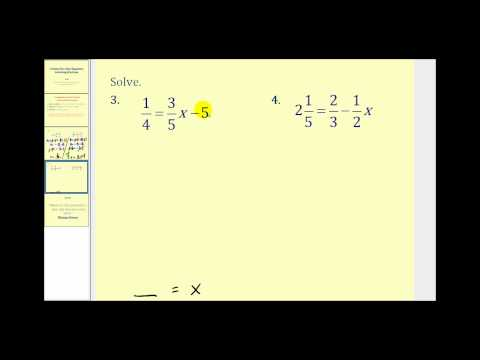## Equation

Subject: Compulsory Maths

#### Overview

The mathematical statement, which cannot be predicted as true or false statements (until the variable is replaced by any number) are known as an open statement.The open statement containing 'equal to' (=) sign and can be true only for a fixed value of variable is called equation.

#### Open Statement

The mathematical statement, which cannot be predicted as true or false statements (until the variable is replaced by any number) are known as an open statement.

x>5, x+3<4, 2y-1≥9 etc. are the example of an open statement.

Linear equations in one variable

The open statement containing 'equal to' (=) sign and can be true only for a fixed value of variable is called equation.Further more in x+2 = 5, the equation has only one variable which is x. So, it is the equation of one variable. Also the variable x has power 1.So it is called a linear equation. Thus, x+2 = 5 is a linear equation in one variable.

Solution to equations

Suppose x+2 = 7

This equation can be true only for a fixed value of x which is 5. So, 5 is called the solution (or root) of the equation.The process of getting a solution to an equation. The process of getting a solution to an equation is called solving equation.

Applications of equations

Generally, we use equations to find the unknown value of any quantity. For this, we should consider the unknown value of the given verbal problems as the variable like x, y, a, b etc. Then the verbal problems should be translated into mathematical sentences in the form of equations. And by solving the equations we obtain the required values.

##### Things to remember
•  The process of getting a solution to an equation.
• The process of getting a solution to an equation is called solving equation.

• The verbal problems should be translated into mathematical sentences in the form of equations.

• It includes every relationship which established among the people.
• There can be more than one community in a society. Community smaller than society.
• It is a network of social relationships which cannot see or touched.
• common interests and common objectives are not necessary for society.
##### Videos for Equation##### Solving Two Step Equations Involving Fractions

Solution:

or, x + 3 = 5
or, x = 5 - 3
∴ x = 2

Solution:

or, x - 2 = 10
or, x = 10 + 2
∴ x = 12

Solution:

or, 5x = 15
or, x = $\frac{15}{5}$
∴  x = 3

Solution:

or, $\frac{x}{2}$ = 7
or, x = 7 × 2
∴ x = 14

Solution:

or, 4(3 - x) = 2x - 15
or, 12 - 4x = 2x - 15
or, -4x - 2x = -15 - 12
or, -6x = -27
or, x = $\frac{27}{6}$
∴ x = $\frac{9}{2}$

Solution:

or, $\frac{3x - 5}{3}$ + x = $\frac{5}{6}$ - $\frac{2x + 3}{2}$
or,  $\frac{3x - 5 + 3x}{3}$ = $\frac{5 - 3(2x + 3)}{6}$
or, $\frac{6x - 5}{3}$ = $\frac{5 - 6x - 9}{6}$
or,  $\frac{6x - 5}{3}$ = $\frac{-4 - 6x}{6}$
or, 6(6x - 5) = 3 (-4 - 6x)
or, 36x - 30 = -12 - 18x
or, 36x + 18x = -12 + 30
or, 54x = 18
or, x = $\frac{18}{54}$
∴ x = $\frac{1}{3}$

Solution:

or, x + 20% of x = Rs 180
or, x + $\frac{20}{100}$ × x = Rs 180
or, x + $\frac{x}{5}$  = Rs 180
or, $\frac{5x + x}{5}$ = Rs 180
or, $\frac{6x}{5}$ = rs Rs 180
or, 6x = 5 × Rs 180
or, x = Rs $\frac{900}{6}$
or, x = Rs 150
∴ x = Rs 150

Solution:

Let the other number be x.
Now,
or, x + 21 = 35
or, x = 35 - 21
or, x = 14
So, the required number is 14.

Solution:

Let the smaller even number be x.
Then, the second consecutive even number = x + 2
And, the third consecutive even number = x + 4
Now,
or, x + (x + 2) + (x + 4) = 30
or, 3x + 6 = 30
or, 3x = 30 - 6
or, x = $\frac{24}{3}$
or, x = 8
∴ The first even number = x = 8
The secomd even number = x + 2 = 8 + 2 = 10
Th e third even number = x + 4 = 8 + 4 = 12
So, the reuired consecutive even numbers are 8, 10 and 12.

Solution:

Let the smaller part of the sum be Rs x.
Then,
the greater part of the sum = Rs (x + 10)
Now,
or, x + (x + 10) = Rs 50
or, 2x = Rs (50 - 10)
or, x = Rs $\frac{40}{2}$
or, x = Rs 20
∴ The smaller part of the sum = x = Rs 20
The greater part of the sum = Rs (x + 10) = Rs (20 + 10) = Rs 30